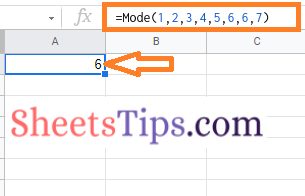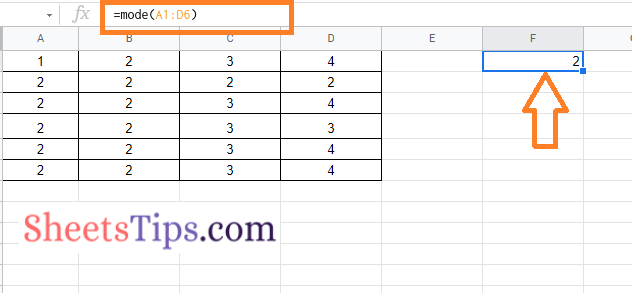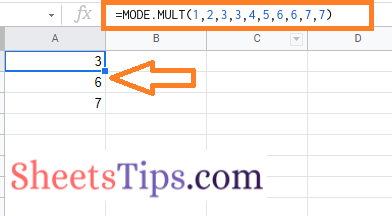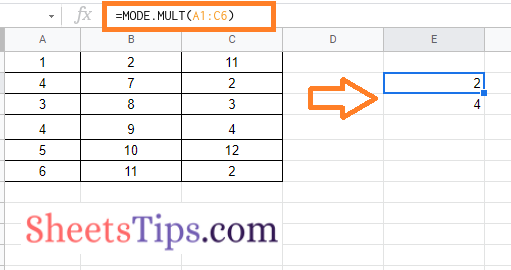# How to Use MODE Function in Google Sheets? (Understand MODE & MODE.MULT Function)

## What is the MODE Function in Google Sheets?

Google Sheets Mode functions are used to find the most frequently occurring number in the Spreadsheet. For example, for the dataset {1,2,3,4,4,5} the mode number is 4 since it occurs more frequently in the dataset.

So basically Google Sheets MODE function is used to find out the most frequently occurring number in the dataset.

Now you would have got a question “How do you find the most frequently occurring text in Google Sheets?”. The answer to this question is the MODE function. Google Sheets MODE function is used to find both frequently occurring text as well as numbers which means Values.

### Understanding Mode Function

The Syntax of MODE functions is =MODE(<Value_1>,[< Value_2>,…])

Let us understand how to use this function syntax in Google Sheets by following the steps given below:

• 2nd Step: Now move to the cell where you want to apply the MODE function syntax.
• 3rd Step: Type the formula =MODE(<Value_1>,[< Value_2>,…]). In this article, we are typing =Mode(1,2,3,4,5,6,6,7).
• 4th Step: Press the “Enter” button and you will see the results as shown below.Since number six is the frequently occurring number in the given dataset, the value 6 is returned.

This is a manual process to use the MODE function in the Google Spreadsheet. In some instances, we might have a huge amount of data for which we need to find the frequently occurring value. In those cases, we can simply replace values with cell ranges. Let us start learning how to do this in the next section of this page.

### How To Find Most Common Values in Google Sheets using MODE Function?

To find the most frequently occurring values in Google Sheets, follow these steps:

• 2nd Step: Now on the homepage, select the dataset for which you want to find the frequently occurring values.
• 3rd Step: Move to the cell where you want to get the results of frequently occurring values,
• 4th Step: Type the formula “=Mode(Cell_Range)”. In this example, we are using the cell range from A1 to D7. So the formula will be “=Mode(a1:d6)“.
• 5th Step: Press the “Enter” button and you will see the results.In this dataset, we find more than values are frequently occurring. However, when we applied the Mode function in the previous example, the Google Sheets showed only the first occurring value by ignoring all the other possible occurrences. So if you want the mode function to show more than one occurrence, then you will have to use the MODE. MULT function.### How to Find Second Most Frequent Text in Google Sheets using MODE.MULT?

To find the second most occurrences in Google Sheets, we will have to use the MODE.MULT function. The steps to use the MODE. MULT function in Google Sheets are explained below: